## Additional results in the Calculations application

When the result is an integer, the calculator gives the following additional results: hexadecimal, binary and prime factorization when relevant.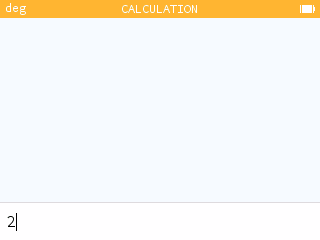When the result is a fraction, the calculator gives the following additional results: mixed fraction and Euclidean division of the numerator by the denominator.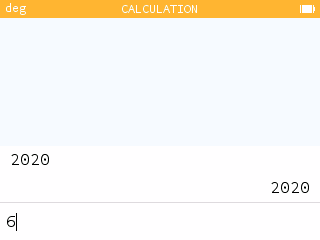When the result or entry is in the form `sin(x)` or `cos(x)`, the calculator gives the following additional results: angle, cosine and sine values and a unit circle representation.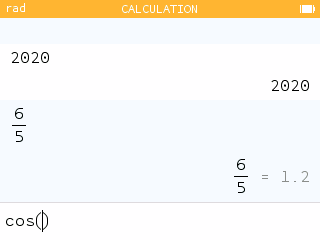When the result is a complex number, the calculator gives the following additional results: modulus, argument, real part, imaginary part and a representation on the complex plane.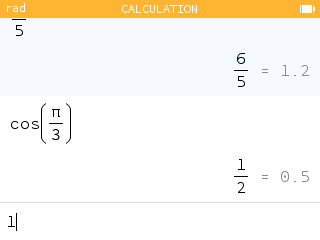## Units in the Calculation application

You can now use units in your calculations. The units are prefixed by the symbol `_`.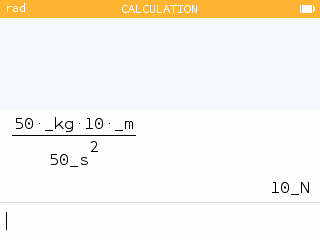The Units section of the Toolbox menu lists all the available units.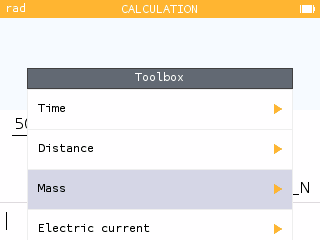The result of the calculation is displayed with the most relevant unit possible.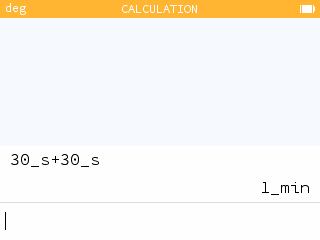To carry out your conversions, use the arrow of the function `sto →` which you can insert by pressing shift and .## Many new features in the Python application

You can select part of a text by holding down the shift key and using the Left Arrow or Right Arrow keys to then be able to copy and paste it.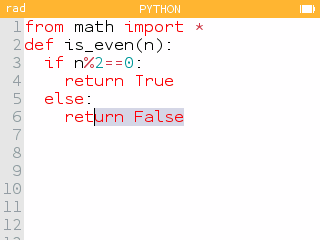It is now possible to use functions from `matplotlib.pyplot` in Python. This will give you access to a tool for drawing curves, scatterplots and histograms.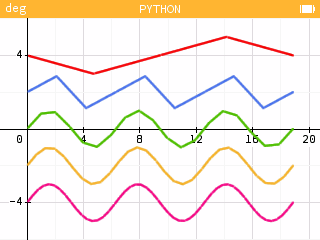Use the new module `ion` to program your games: the function `keydown()` indicates whether the key in argument is pressed or not. Click here to see a sample script.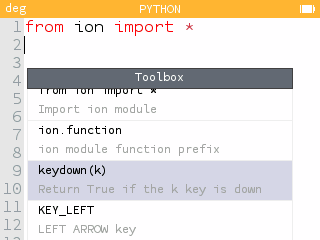For more readability, you can choose the font size used in Python by going to the Settings application.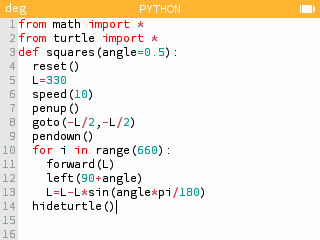Also take advantage of twice the storage space for your Python scripts.

## And also...

VoYou can now use the Fisher distribution in the Probability application.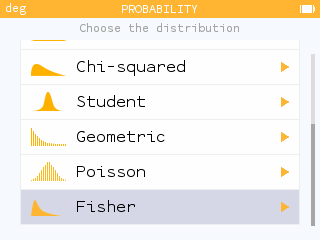The Equations application displays a message when user-created variables are ignored.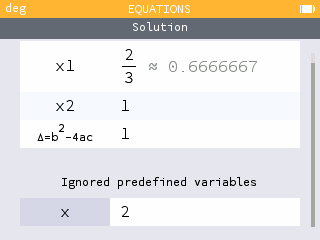When exam mode is activated, the Exam Mode menu in the Settings application explains how to exit exam mode.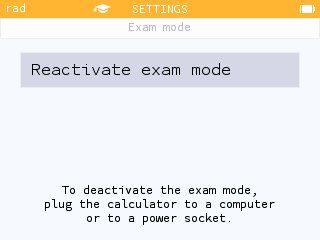• The model used for power and trigonometric regressions is now consistent with that used in other math software.
• The movement of the cursor on a curve becomes faster if the arrow key is pressed for a long time.
• Scrolling through tables of values is faster.
• Expressions of the type `y(x)` are now understood as multiplications when y has not been defined as a function.
• The following features have been added to the catalog menu of the Toolbox in the Python application: `list.append(x)`, `list.clear()`, `list.count(x)`, `list.index(x)`, `list.insert(i,x)`, `list.pop(i)`, `list.remove(x)`, `list.reverse()` and `list.sort()`.
• A sample script `parabola.py` has been added to the Python application scipts list. To draw the figure, run the command `simulation()`.

## Special thanks

We would like to thank the contributors who helped develop this update: Alexandre André, Adrien Bertrand, Jean-Baptiste Boric, Lionel Debroux, Joachim Le Fournis and Neven Sajko.